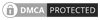## Bernoullis Theorem

Bernoulli's theorem is a method of expressing the law of conservation of energy to the flow of fluids.Bernoulli's principle stats that, in the flow of fluid (a liquid or gas), an increase in velocity occurs simultaneously with decrease in pressure. That statement is a simplification of Bernoulli's equation (below) which plots the situation at any point on a streamline of the fluid flow and applies the law of conservation of energy to flow. Put another way, the total energy of the flow at any point along a horizontal pipe is equal to the sum of the pressure head, the velocity head and the elevation in the absence of friction. This is a principle of considerable importance to those concerned with flow in sprinkler pipework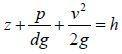When

z = Potential head or elevation
p = Pressure
v = Velocity
g = Acceleration of gravity
d = Density of fluid

If friction losses are ignored and no energy is added or removed from the pipe the total head h, in the above equation will be constant for any point in the fluid. However in practice energy will increase and decrease with the effect of pumps and friction loss and this must be included in the Bernoulli's equation. All practical formulas for the flow of fluids are derived from the Bernoulli's theorem with modifications to account for losses due to friction.

## Fire Sprinkler Defenitions

### A

Area of operation

The maximum area over which it is assumed that the sprinkler will operate and is some time called the "assumed maximum area of operation"

Barometer

An instrument used for the measurement of atmospheric pressure

Bar

Is a unit of pressure 1 bar = 10000 pascal (Pa).  The bar is used through Europe for the measurement of pressure in fire sprinkler systems.

Bernoulli's equation

A mathematical expression of the principle of conservation of energy

Booster pump

A fire pump used to boot the pressure of the existing water supply

Bourdon gauge

The most common device used to measure system pressures.

Centrifugal pump

Modern industrial fire pumps are centrifugal pumps.  Pressure is added to the water by the centrifugal force created by a rotating wheel (impeller) or the rotating vanes of a turbine.

### B

Circulation relief valve

A small relief valve that opens up and provides enough water flow into and out of the pump to prevent the pump from overheating when it is operating at churn against a closed system.

Complex loop

A piping system that is sometimes called a "grid" and is characterised by one or more of the following:  more than one inflow point, more than one outflow point, and/ or more than two paths between inflow and outflow points.

### H

Hardy cross method

An interactive technique used for solving the complicated problems involving gridded water supply systems.

Hazen-Williams formula

An empirical formula for calculating friction loss in water systems that is the fire protection industry standard.  To comply with the most nationally recognised standards, the Hazen-Williams formula must be used.

Pressure expressed in units of Metre of water.

Horizontal split -case pump

A centrifugal pump with the impeller shaft installed horizontally and often referred to as a split-case pump.  This is because the case in which the shaft and impeller rotates is split in the middle and can be separated exposing the shaft, bearings and impeller.

Hydraulics

The branch of fluid mechanics dealing with the mechanical properties of liquids (in the text water) and the application of these properties in engineering.

Hydrokinetics

A branch of hydraulics having to do with liquids (water) in motion, particularly in relation to forces created by or applied to the liquid in motion

Hydrostatics

A branch of hydraulics dealing with the properties of liquids (water) at rest, particularly in relation to pressures resulting from or applied to the static liquid.

Hydraulically most favorable area

Area of operation for which the water flow is at its maximum for a specified pressure, measured at the main control valve or pump set.

hydraulically most unfavorable area

Area of operation for which the system pressure, when measured at the main control valve or pump set, is required to be at its maximum to achieve the specified design density

### J

Jockey pump

A jockey pump is a small capacity, high pressure pump used to maintain constant pressures on the fire protection system.  A jockey pump is often used to prevent the main pump from starting unnecessarily.

### K

Kinematic viscosity

The kinematic viscosity of a fluid is the ration of its absolute viscosity to its mass density.

Kinetic energy

The energy which a body possesses because of its motion.

### L

Laminar flow

A fluid is in the state of laminar flow if its Reynolds number is 2,100 or less; laminar flow is related to very low liquid velocities.

### M

Maximum flow demand

The flow discharge from the sprinkler heads located at the hydranliclly most favourable area when balanced to a fire pumps flow / pressuer curve (QMAX point)

Moody diagram

A Diagram used with the Darcy-Weisbach friction loss computation technique to relate the Reynolds number, pipe size, and roughness to a friction factor

### N

Net pressure

The net pressure is the pressure added to the system  by the pump.

### O

Orifice plate meter

An orifice plate meter is a device used for measuring water flow and is similar in principle to a Venturi meter.  The change of water velocity is accomplished by using a plate with an orifice that is smaller than the diameter of the pipe in which it is placed.

### P

Pascal's law

Principle 1, known as Pascal's law, points out that pressure acts in all directions and not simply downward.

Pascal

The SI unit for pressure is the pascal (Pa) which is equal to one Newton per square meter (N/m2).  For fire protection this measurement of pressure is small so the unit Bar or kPa is used in most part of the world.

Piezometer tube

This device uses the heights of liquid columns to illustrate the pressures existing in hydraulic systems.

Pitot tube

A common device used to measure velocity pressure and thus fluid velocity.  The pitot tube consists of a small diameter tube, usually about a one-sixteenth inch in internal diameter which is connected to a pressure gauge.

Potential energy

Stored energy which has the ability to perform work once released.

Pressure

is the force per unit area (symbol P).

Is a term used in fluid mechanics to represent the internal energy of a fluid due to the pressure exerted on its container. It may also be called static pressure head or simply static head.

PSI

In fire protection, pressure is most often dealt within units of pounds per square inch (psi).

### R

Relief valve

The relief valve is provided to open up and discharge water to a drain should the pressure become excessive.  This valve is located between the pump and the discharge check valve and is required with pumps driven by variable speed drivers.

Residual pressure

The pressure at the test hydrant while water is flowing.  It represents the pressure remaining in the system while the test water is flowing.

Reynolds number

is a dimensionless number that state if the flow is in a laminar or turbulent stat (Symbol Re) .

### S

Simple loop

A loop in which there is exactly one inflow point and one outflow point, and exactly two paths between the inflow and outflow points.

Specific gravity

The specific gravity (Sg) of a substance may be defined generally as the ratio of the weight density of the substance to the weight density of another substance, usually water.

Static pressure

The normal pressure existing on a system before the flow hydrant is opened.

### T

Total energy

The total energy (TE) at any point in a system might be defined as the sum of the potential energy and kinetic energy at that point.

Turbulent state

Fluid flow is in the turbulent state higher velocities where there is no definite pattern to the direction of the water particles.  Turbulent flow is reflected by a calculated Reynolds number in excess of 2,100.

### V

Venturi meter

When coupled with a differential manometer, a venture meter may be used to measure water velocity.  The device consists essentially of a piece of pipe in which the cross-sectional area has been constricted.

### W

Water hammer

Stopping any flowing stream too rapidly can cause a phenomenon called water hammer.  Water hammer is a violent increase in pressure which can be large enough to rupture the piping.

## Fire sprinkler hydraulic calculations and design density

In fire sprinkler design one of the most important concepts is the principal of water density yet many fire sprinkler system design engineers do not fully understand the concept, this sort instruction will hopefully full fill this requirement.

We often refer to Design Density in lazy preference to Design Density of Discharge which, in turn, is a short way of saying Density of Application of Water. This is an unusual use of the word 'density' since we know, of course, that the density of water is 1. The density of application, however, means how much water we apply over a certain area, much the same as pressure is a force applied over a unit area.

We are, therefore talking about a volume of water spread over a certain area in a unit of time.

Volume can be measured in litre
Area can be measured in m2
Time can be measured in minutes

The density of water application would be measured thus:

Volume / Area x Time or Litre / m3 x min

It is necessary to bring this formula to a manageable state by changing the units. As a Litre of water is defined as cubic decimetre which is 10 centimetres × 10 centimetres × 10 centimetres, (1 L ≡ 1 dm3 ≡ 1000 cm3). Hence 1 L ≡ 0.001 m3 ≡ 1000 cm3 and 1 m3 (i.e. a cubic metre, which is the S.I. unit for volume) is exactly 1000 L.

Therefore, we can rewrite the formula:

dm x dm x dm / 10dm x 10dm x min

This can be simplified by cancelling out

dm / 100min = 100mm / 100min = 1mm / 1min = mm/min

The density of application can, therefore, be measured in millimetres per minute (mm/min).

Whichever route you take, it is important to realise that when we use this strange, apparently linear unit, we are talking about a volume of water discharged over an area of 1m2 in 1 min.

In the case of Ordinary Hazard Installations (EN 12845) with a Density of Discharge of 5.0 mm/min bearing in mind that this really means 5 L/min2/min then we are applying less than half a bucket full of water on every square metre each minute.

The art, of course, is how you tell it or, in this case, how you apply it. The sprinkler head distributes the water in an even pattern so that in the case of OH3, each of the 12m2 covered by the head receives its share of water. When testing a sprinkler head the floor is covered with 1m2 trays and after a discharge for 1 minute, there should be water in each tray to a depth of 5mm. The volume of water in the tray would be 5mm x 1000mm x 1000mm = 5,000,000mm3. Since there are (100 x 100 x 100) ie. 1,000,000mm3 in 1dm3 the volume of water will, of course, be 5dm3 or 5 litres.

Taking OH3 as an example, if we design for a maximum of 18 sprinkler heads operating each capable of covering 12m2, then the maximum area of operation will be (18 x 12)m2 = 216m2.  If each of the 18 sprinkler heads is discharging 5dm3/m2 every minute then we will require a flow of (5 x 18 x 12)dm3/min = 1080dm3/min. In calculating pipe sizes this is approximated to 1000dm3/min.

Now we know the theory of 'Design Density' we can use it in are fire sprinkler hydraulic calculations to find the quantity of water required to flow from a fire sprinkler. If we know the area a sprinkler head is covering and the required design density then we can use the following formula:

Area x Density = Quantity

Therefore if we have a fire sprinkler head which is covering 8m2 and we require 12.5 mm/min

8m2 x 12.5 mm/min = 100 Litres/min

This would be the minimum flow rate required for the sprinkler head to prove the correct Design Density.  The specific design density to be used for design purposes is determined by reference to the occupancy fire hazard of the building once this is known the applicable design standard such as EN 12845, BS 9251 or NFPA 13 will have tables of occupancies from which you can find the required design density.

### A practical design density example

Let’s take an example, if we are designing a fire sprinkler system and we know that we required a design density of 15.0 mm/min over the design area then this is the starting point for our fire sprinkler hydraulic calculation.

We now need to take the first most remote fire sprinkler on the branch line (range pipe) and find the actual area the fire sprinkler is covering let’s assume it’s 7.5m2 for this example. We can now calculate the minimum flow rate from the fire sprinkler to provide the required design density, this can be found from the equation:

Q = D x A

When:

Q = flow rate from the fire sprinkler
D = Design density
A = Area of coverage for the fire sprinkler

For our example:

15 mm/min x 7.5m2 = 112.50 L/min

We now know that the most remote fire sprinkler must have a flow rate which is equal to or greater than than 112.50 L/min.

The next step in the calculation is to check that we can get this flow rate from the fire sprinkler and what pressure will be required.

We hope you have found this short introduction to water design density to be informative and that it will help you understated one of the fundamental concepts of sprinkler system design.

##### Footnotes:

The litre is the SI derived unit for volume which is the volume of a cube with 10 cm sides and has the symbol L or l. A decimeter (dm3) is 1,000 cubic centimetre(cm3) or 1/1000 of a cubic metre.

## Glossary of hydraulics for fire protection

A B C D E F G H I J K L M N O P Q R S T U V W X Y Z

A

• Area of operation: The maximum area over which it is assumed that the sprinkler will operate and is some time called the "assumed maximum area of operation"

B

• Barometer: An instrument used for the measurement of atmospheric pressure

• Bar: Is a unit of pressure 1 bar = 10000 pascal (Pa).  The bar is used through Europe for the measurement of pressure in fire sprinkler systems.
• Booster pump: A fire pump used to boot the pressure of the existing water supply
• Bourdon gauge: The most common device used to measure system pressures.

C

•  Centrifugal pump: Modern industrial fire pumps are centrifugal pumps.  Pressure is added to the water by the centrifugal force created by a rotating wheel (impeller) or the rotating vanes of a turbine.
• Certified shop test valve: Before a pump is shipped by the manufacturer, it will be tested in the shop.  The results of this test will be plotted on graph paper.
• Circulation relief valve: A small relief valve that opens up and provides enough water flow into and out of the pump to prevent the pump from overheating when it is operating at churn against a closed system.
• Complex loop: A piping system that is sometimes called a "grid" and is characterised by one or more of the following:  more than one inflow point, more than one outflow point, and/ or more than two paths between inflow and outflow points.
• Controller:  The electric control panel used to switch pump on and off and to control its operation.

D

• Darcy-Weisbach:  Technique used to establish the pressure lost to friction in a piping system.

• Desing Density: The density of discharge is given as mm/min and is the minimum amount of water which is needed over the design area (or sprinkler head) for each minute of operation. The density of discard require of any risk is dependent on the occupancies, classification of goods and the design standard been applied.

• Desing Point: A point in the distribution pipe of a precalculated installation from where the pipework is sized by hydraulic calculation
• Differential manometer: A device whose primary application is to reflect the differences in pressures between two points in a system.

F

• Flow hydrant: The hydrant from which the water is discharged during a hydrant flow test.
• Flow test:  Tests conducted to establish the capabilities of water supply systems and referred to as flow tests because they involve flowing fire hydrants.  The objective of a flow test is to establish quantity ( gallons per minute) and pressures available at a specific location on a particular water supply system.
• Fluid:  Any substance that can flow; a substance which has definite mass and volume at constant temperature and pressure, but no definite shape; and with the inability to sustain shear stresses.
• Fluid mechanics: In the general terms of physics, force is that which causes motion.

• Force: In the general terms of Physics, force is that which causes motion.

G

H

• Hardy cross method: An interactive technique used for solving the complicated problems involving gridded water supply systems.
• Hazen-Williams formula: An empirical formula for calculating friction loss in water systems that is the fire protection industry standard.  To comply with the most nationally recognised standards, the Hazen-Williams formula must be used.
• Head: Pressure expressed in units of Metre of water.
• Horizontal split -case pump: A centrifugal pump with the impeller shaft installed horizontally and often referred to as a split-case pump.  This is because the case in which the shaft and impeller rotates is split in the middle and can be separated exposing the shaft, bearings and impeller.
• Hydraulics: The branch of fluid mechanics dealing with the mechanical properties of liquids (in the text water) and the application of these properties in engineering.
• Hydrokinetics: A branch of hydraulics having to do with liquids (water) in motion, particularly in relation to forces created by or applied to the liquid in motion.
• Hydrostatics: A branch of hydraulics dealing with the properties of liquids (water) at rest, particularly in relation to pressures resulting from or applied to the static liquid.

• Hydraulically most favorable area: Area of operation for which the water flow is at its maximum for a specified pressure, measured at the main control valve or pump set

• hydraulically most unfavorable area: Area of operation for which the system pressure, when measured at the main control valve or pump set, is required to be at its maximum to achieve the specified design density

J

• Jockey pump: A jockey pump is a small capacity, high pressure pump used to maintain constant pressures on the fire protection system.  A jockey pump is often used to prevent the main pump from starting unnecessarily.

K

• Kinematic viscosity:  The kinematic viscosity of a fluid is the ration of its absolute viscosity (lb sec/ft2) to its mass density (lb sec2/ft4).
• Kinetic energy: The energy which a body possesses because of its motion.

L

• Laminar flow:  A fluid is in the state of laminar flow if its Reynolds number is 2,100 or less; laminar flow is related to very low liquid velocities.
• Liquid:  A fluid having a definite volume, unlike gases, which will expand to fill the vessel containing it.

M

• Maximum flow demand: The flow discharge from the sprinkler heads located at the hydranliclly most favourable area when balanced to a fire pumps flow / pressuer curve (QMAX point)

• Moody diagram: A Diagram used with the Darcy-Weisbach friction loss computation technique to relate the Reynolds number, pipe size, and roughness to a friction factor.

N

• Net pressure:  The net pressure is the pressure added to the system  by the pump.

O

• Orifice plate meter:  An orifice plate meter is a device used for measuring water flow and is similar in principle to a Venturi meter.  The change of water velocity is accomplished by using a plate with an orifice that is smaller than the diameter of the pipe in which it is placed.

P

•  Pascal's law:  Principle 1, known as Pascal's law, points out that pressure acts in all directions and not simply downward.
• Pascal: The SI unit for pressure is the pascal (Pa) which is equal to one Newton per square meter (N/m2).  For fire protection this measurement of pressure is small so the unit Bar or kPa is used in most part of the world.

• Piezometer tube: This device uses the heights of liquid columns to illustrate the pressures existing in hydraulic systems.
• Pitot tube:  Common device used to measure velocity pressure and thus fluid velocity.  The pitot tube consists of a small diameter tube, usually about one-sixteenth inch in internal diameter which is connected to a pressure gauge.
• Potential energy: Stored energy which has the ability to perform work once released.
• Pressure: is the force per unit area (symbol P).
• Pressure head: Is a term used in fluid mechanics to represent the internal energy of a fluid due to the pressure exerted on its container. It may also be called static pressure head or simply static head.
• PSI: In fire protection, pressure is most often dealt within units of pounds per square inch (psi).

R

• Relief valve: The relief valve is provided to open up and discharge water to a drain should the pressure become excessive.  This valve is located between the pump and the discharge check valve and is required with pumps driven by variable speed drivers.
• Residual pressure:  The pressure at the test hydrant while water is flowing.  It represents the pressure remaining in the system while the test water is flowing.
• Reynolds number: is a dimensionless number that state if the flow is in a laminar or turbulent stat (Symbol Re) .

S

• Simple loop:  A loop in which there is exactly one inflow point and one outflow point, and exactly two paths between the inflow and outflow points.
• Specific gravity: The specific gravity (Sg) of a substance may be defined generally as the ratio of the weight density of the substance to the weight density of another substance, usually water.
• Static pressure:   The normal pressure existing on a system before the flow hydrant is opened.

T

• Total energy:  The total energy (TE) at any point in a system might be defined as the sum of the potential energy and kinetic energy at that point.
• Turbulent state:  Fluid flow is in the turbulent state higher velocities where there is no definite pattern to the direction of the water particles.  Turbulent flow is reflected by a calculated Reynolds number in excess of 2,100.

V

• Venturi meter:  When coupled with a differential manometer, a venture meter may be used to measure water velocity.  The device consists essentially of a piece of pipe in which the cross-sectional area has been constricted.

W

• Water hammer:  Stopping any flowing stream too rapidly can cause a phenomenon called water hammer.  Water hammer is a violent increase in pressure which can be large enough to rupture the piping.

## Hazen Williams formula for use in fire sprinkler systems

The Hazen Williams formula is an empirical equation and has long been used for calculating the friction loss in pipework for water based fire sprinkler protection systems. This equation uses the coefficient C to specify the pipes roughness, which is not based on a function of the Reynolds number, as in other pressure loss equations. This however has the disadvantage that the equation can only be used when water is flowing within the 'turbulent' flow range. If the system is outside the normal pressure and flow range or the system is to use additives, or will be subject to unusual temperature conditions then the Darcy Weisbach equation may be more appropriate.

Gardner Williams and Allen Hazen first started there Experimentation in the early part of the 20th century.  They began to record the friction loss through pipe in numerous experimentations in their studies resulted in the development of a empirical formula which we know today as the Hazen- Williams formula.  The formula along with a series of friction loss tables computed from the formula was published in 1903.

The Hazen Williams formula has the advantage of been simple to calculate by using a scientific calculator where as the Darcy Weisbach equation requires the use 'f' friction factor and this can only be calculated by an number of iterations as 'f' is on both sides of the equation. You can use a Moody diagram to find the value of 'f' however this is both time consuming and almost certainly not an inaccurate method.

The Hazen William formula has now become adopted through the world as the pressure loss formula to use for the hydraulic design of fire sprinkler systems and in almost all cases the use of the hazen william formula will provide adequate answers. The Hazen William formula can also be used for the calculation of water mist systems where the system pressure does not exceed 12 bar (low pressure water mist systems) or the water velocity does not exceed 7.6 m/s and the minimum pipe size is 20mm in the case of intermediate and high pressure water mist systems.

You can use Canute Hcalc hydraulic calculator or our online Hazen Williams calculator to visually explore the relationship between the flow, pipe diameter and the pipe C-Factor in the Hazen Williams formula which will give you a good understanding of the formula. The Hcalc software is free to download and use.when:

p = pressure loss in bar per meter
Q = flow through the pipe in L/min
C = friction loss coefficient
d = internal diameter of the pipe in mm

You can seen the above equation that if Q is raised to the power of 1.85 in the above equation this has the effect that if the flow is doubled and all other things remain constant the friction loss will increase by almost 4 times, if the flow was to triple the friction loss would almost be 9 times greater.  You can also see that the pipe diameter D is raised to the power of 4.87 and that it's in the denominator on the right hand side of the equation.  Therefore any increase in the pipe size will reduce the friction loss if all other factors remain the same.  If the diameters doubled, the friction loss will be reduced by almost a factor of 1/32 likewise if the pipe diameter is tripled The friction loss would be reduced to about 1/243 of its original value.

The Hayes-Williams formula which is empirical yields only approximate results however it is considered to be accurate enough to be used for the calculation of fire sprinkler systems and indeed the formula is stipulated in both NFPA 13 and EN 12845.  There are certain cases such as high pressure water mist where the use of Darcy-Weigbach formula would be more appropriate and this is set out in NFPA 750.

## Value of C for use in the Hazen-Williams formula

Listed in the table bellow are typical values for the coefficient C, which can be used in the Hazen-Williams formula for different fire sprinkler design standards. The value of C represents the pipes roughness with higher values of C giving lower friction losses. The values given in the design standards allow for degradation of the pipe, for instance new cast iron pipe has a C coefficient of 130 and EN 12845 gives the value of 100, this is equivalent to a pipe, which is about 20 years old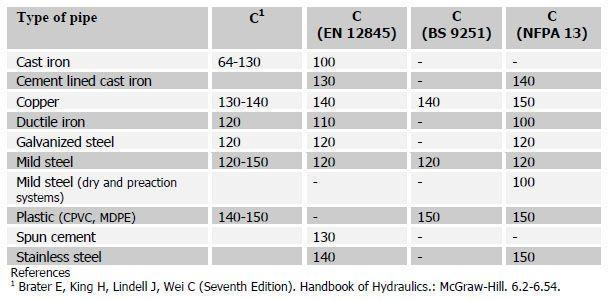## The long way - by hand

In this article, will demonstrate some of the basics for carrying out fire sprinkler calculations by the long hand method with just the aid of a scientific calculator or our own hydraulic calculator - Hcal2 which you can freely download from our website.

We will for this example use simple three sprinklers and three pipes which would of course be part of a much larger fire sprinkler system. These basic procedures can also be used for calculating many other types of systems such as fire hydrant, hose reel or the discharge from a water cannon or monitor we can also use the same principal for almost all other water-based fire protection systems if we have a k-factor for the output device (fire sprinkler, water mist nozzle and so on).

In this example, will we use a very simple system with just three sprinklers and three pipes this is often called a range pipe or branch pipe, which is part of a larger 'tree system'. A tree system is 'end feed', that is water is only fed from one direction as opposed to a grid or loop system when water may arrive at the sprinkler head from more than one direction.

Below is a diagram of the three sprinklers and three pipes which we will calculate.  We have dimensioned the pipe lengths and given each junction point a unique node reference number which we use throughout the calculations.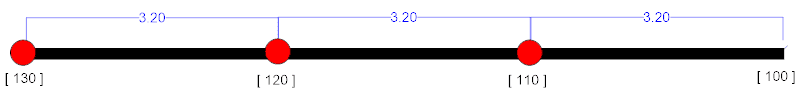For each pipe, we need to know the pipe length, internal diameter (ID) of the pipe and the pipe material so we can determine the pipes c-factor, the table below summarises the pipe data which we will need for the calculation for this example:

 Node Ref Pipe Size ID (mm) Length (m) C-factor 130-120 27.30 3.20 120 120-110 27.30 3.20 120 110-100 36.00 3.20 120

We will also we will need some additional information such as the type sprinkler head, the area each head is covering, the design density for each sprinkler head in the system.

For this example, we will use the following design parameters:

design density: 7.50 mm/min
sprinkler head: K-factor of 70 with a minimum pressure 0.5 bar

In this example, we have kept it very simple and used the same sprinkler head for all three sprinklers but this may not always be the case so again it may be useful to summarises the information in a table such as this:

 Node Ref Design Density(mm/min) Sprinkler k-factor Sprinkler minimumpressure (Bar) Head area (m2) 130 7.50 70 0.5 10.20 120 7.50 70 0.5 10.20 110 7.5 70 0.5 10.20

The first step is to calculate the minimum flow which will be required at the most remote sprinkler which in this case is at node , this is a two-step process as will need to calculate the minimum flow required to satisfy the 7.50 mm/min design density and then find the flow rate from the sprinkler given the sprinklers minimum pressure requirement, whichever is the greater flow will become our initial flow from the first sprinkler at node .

We will first calculate the flow given the design density of 7.50 mm/min and the area the head is covering, we do this by multiplying the design density by the head area:

Equation 1:

q1 = (design density) x (area per sprinkler)

In this example, this gives:

q1 = 7.50 mm/min x 10.20 m2 = 76.50 L/min

The second step is to calculate the minimum flow from the sprinkler given the K-Factor and the minimum head pressure by using the standard K-Factor formula:

Equation 2:

q = kp0.5

Where

p = the required pressure
q = the required flow from the first sprinkler
k = the discharge coefficient of the sprinkler (k-factor)

In this example, this gives:

q = 70 x 0.50.5 = 49.50 L/min

By comparing the two calculations above we can see that the minimum flow required from the sprinkler head will be 76.50 L/min as this is the highest flow rate from the two calculations and is required to meet the 7.50 mm/min design density.  We can also see that the minimum sprinkler pressure of 0.5 bar is not sufficient to produce the required flow rate so the next step will be to determine what pressure will be required to produce the required flow of 76.50 L/min at the first sprinkler head at node  we can do this by using equation 3.

Equation 3

p = (q/k)2

In the example, this gives:

p = (76.50 / 70)0.5 = 1.194 bar

We have now determined the minimum pressure and flow for the first sprinkler at node  which will be 76.50 L/min @ 1.19 bar the next step is to calculate the pressure drop in the pipe between node  and  and for this we will use the Hazen-Williams pressure loss formula.

Equation 4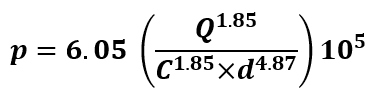Where

p = pressure loss in bar per meter
Q = flow through the pipe in L/min
C = friction loss coefficient
d = internal diameter of the pipe in mm

We know that the flow rate from the sprinkler at node  is 76.50 L/min and this will be the flow rate in the first pipe between nodes -. As the pipe has an internal diameter of 27.30 mm and has a C value of 120 this will give us: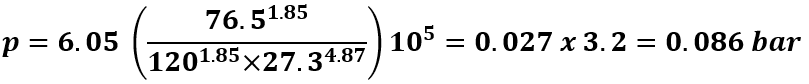The pressure loss in the first pipe is 0.027 Bar/m and the total pressure loss in the pipe is 0.086 bar.

We now need to add the pressure loss in the pipe to the start pressure at the sprinkler head at node  which was 1.19 bar to find to pressure at node  and at the seconded sprinkler head at node  this gives us 1.194 + 0.086 = 1.28 bar.

The next step is to find the flow from the seconded sprinkler head at node  to do this we will use the K-Factor formula

Equation 5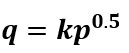This gives 70 x 1.2800.5 = 79.20 L/min from the sprinkler head at node  which we now add to the flow in the first pipe node - to find the total flow in the second pipe - to find the total flow in the seconded pipe which is 155.70 L/min.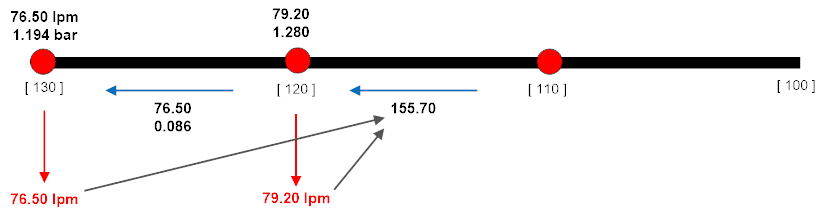Having found the total flow in the seconded pipe - we can now find the pressure loss in, to do this we will use the Hazen-Williams pressure loss, formula 4 which we used above this gives us: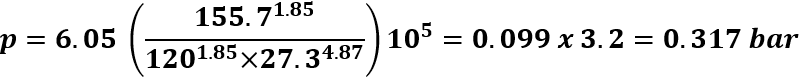We now add the pressure loss 0.317 bar to the pressure at node  to find the pressure at node  this give us: 0.317 + 1.280 = 1.597 bar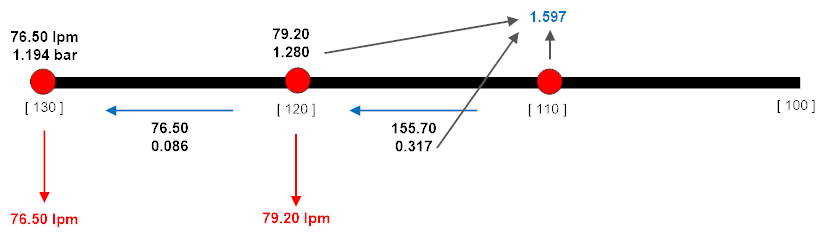We now need to find the flow from the sprinkler at node  we do this by using the k-factor given in equation 5 as we now know the pressure at node  is 1.597 bar, this gives 70 x 1.5970.5 = 88.50 L/min from the sprinkler head at node .  We now add this flow to the flow in the seconded pipe - to find the total flow in the third pipe - which will give us the flow of 244.20 L/min.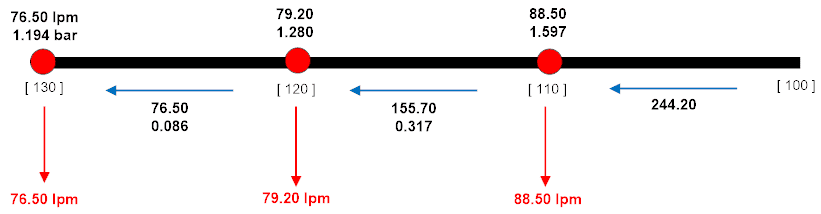The last step is to find the pressure loss in the third pipe - and again we will use the Hazen-Williams pressure loss formula given is formula 4 above. However, the last pipe has an internal diameter of 36.0 mm so this gives us: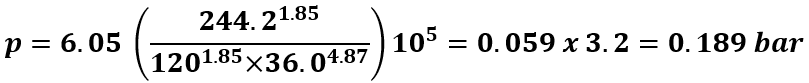We now add the pressure loss in this pipe to the pressure at node  to find the pressure at node  this will be 0.189 + 1.597 = 1.786 bar.  We have now completed the calculation for all three sprinkler heads and have found the source pressure and flow required for this system is:

244.20 L/min @ 1.786 Bar

This pressure and flow is often referred to as the source requirement for the system and is the minimum pressure and flow required for the system for it to be able to provide the required design density (in this example 7.50 mm/min) at the most remote head [MRH] at node .

You should also be able to see that only the Most Remote Head has the minimum requirement of 7.50 mm/min design density and all the other sprinklers will have a higher pressure as they are hydraulically closer to the water source so they will have a higher pressure and will discharge more water through the sprinkler this can be seen in the table below:

 Node Ref min Design Density(mm/min) Pressure (Bar) Flow from sprinkler(L/min) Head Area(m2) ActualDesign Density 130 [MRH] 7.50 1.194 76.50 10.20 7.50 120 7.50 1.280 79.20 10.20 7.76 130 7.50 1.597 88.50 10.20 8.68

Sprinkler calculation step by step

1. Calculate minimum flow from the MRH with the sprinkler minimum pressure and k-factor
2. Calculate the minimum flow given the system design density and sprinkler head area.
3. If the calculation in step 2 is the highest flow demand, then calculate the required head pressure otherwise we can use the minimum sprinkler pressure in step 1.
4. Calculate the pressure loss in the pipe.
5. Add the head pressure to the pressure loss in step 4 to determine the pressure at the next sprinkler.
6. Use the k-factor formula to determine the flow from the sprinkler head.
7. Repeat step 4 to 6 until you do not have any more sprinklers or pipes

## Hydrostatics and hydraulic calculationsHydrostatics is a branch of hydraulics that deals with fluids at rest. As mentioned earlier, water is the single most important component of fire protection and hydraulic calculations, so in this section will look at water at rest.

### Basic Principles

1. The pressure at a point in the liquid is equal in every direction.
2. Pressure applied from an external source on a confined liquid will be transmitted in all directions throughout the liquid without a reduction in magnitude.
3. The pressure created by a liquid in an open container is directly proportional to the depth of the liquid.
4. The pressure created by a liquid in an open container is directly proportional to the density of the liquid.
5. The shape or volume of a liquid has no influence on the pressure created by the liquid.

A common misunderstanding is that pressure always acts in a download direction, this fallacy is fostered by the understanding that pressure is related to weight. Principle 1 which is known as Pascal's Law points out that pressure acts in all directions as shown in figure 1. The four pressure gauges are in different orientations but taking a pressure reading from the same point, all four gauges will show the same pressure.Principle two has obvious practical limitations. The idea of undiminished pressure transmission throughout a confined liquid would be more readily accepted if the incompressible nature of liquids is more fully understood. Gases will contract in volume when placed under pressure, whereas liquids will maintain their volume when placed under pressure. Because the volume is not reduced, the increase in pressure is propagated throughout the entirety of the liquid in every direction with no drop in magnitude.

Principle three is an essential part of fire reduction hydraulics. The pressure created by a column of liquid is directly proportional to the depth of the liquid. Water has a density of 1000kg/m3. Therefore, 1 cubic metre of water will weigh 1000kg.

Principle four is closely linked to principle three in that pressure created by a liquid is also directly proportional to the density. Mercury, for example, has a density that is 13.6 times greater than water, so the pressure created by 1 metre of mercury will be 13.6 times greater than the pressure created by 1 metre of water. Both principle three and four can be summarized by the following relationship:

P=w.h

Where:

• P = Pressure (bar)
• w = specific weight of the liquid in kg/m3.
• h = height of the liquid column in metres.

Principle five is generally the most difficult to understand. Pressure developed is not related to size or shape of the container, only the depth and density of the liquid. Principle five applies to pressure, and not to force. A column of water has the same pressure throughout but will have different weights depending on where the pressure reading is taken. Pressure only depends on the depth of the liquid, and is independent of the shape or volume of the container.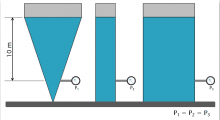It is common to hear fire protection specialists refer to water pressure in terms of head. Head is pressure expressed in units of metres of water rather than bar, and can be expressed in the general form:

h= P/w

Where:

• h = head in metres.
• P = pressure (bar).
• w = the specific weight in kg/m3.

### Buoyancy

Buoyancy is where an object floats on the surface of a liquid. For an object to do this, the force in the downwards direction must be equalised by a force in the upward direction. The force acting downwards will equal the weight density of the material times the volume of the object.

F1=w.V

In order for the object to remain stationary, F1, must also equal w.V. The amount of material that sticks above the surface of the liquid, and if an object will float, depends upon the relationship between the weight densities of the objects and the liquid. A solid object will float in a liquid only if its weight density is less than or equal to that of the liquid. The proportion of a floating object that will sink below the surface of a liquid will depend upon how much of the liquid will equal the weight of the object.

### Potential Energy

It is important to understand the part that hydrostatic pressure plays in the energy composition of a hydraulic system. Hydrostatic pressure composes the potential energy component of the total energy of a hydraulic system. Potential energy is stored energy. It has the ability to perform work once released. It is easy to visualise the potential of a stretched rubber band or a coiled spring. However, elevated or pressurised water also contains potential energy. Because energy is the ability to do work, and work is the product of force times distance and has units of metre-kilograms; potential energy is the potential or ability to move some unit of weight (force) some distance, or:

PE=W.h

Where:

• W = weight in kilograms.
• h = height in metres.Canute LLP
15 Queen Square
Leeds, West Yorkshire
United Kingdom LS2 8AJ

t: +44 (0) 113 328 0350
e: info@canutesoft.com

 Copyright © 2021, Canute LLPRegistered in England & Wales, Partnership No. OC305985